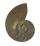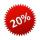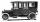# Numbers - math word problems

#### Number of problems found: 644

• When will I be a millionaire?Barry monthly send 280 euros to the bank, which he deposits bear interest of 2.1% p. A. Calculate how many months must Barry save to save 1000000 euros? Inflation, interest rate changes, or bank failures ignore.
• Logarithmic equationSolve equation: log33(3x + 21) = 0
• Percentages and numbersHow many percent is number 426 greater than number 71?
• Car inflationAbout what percentage price of car increased if price rose from €1880 to €2200?

We apologize, but in this category are not a lot of examples.
Do you have an interesting mathematical word problem that you can't solve it? Submit a math problem, and we can try to solve it.

We will send a solution to your e-mail address. Solved examples are also published here. Please enter the e-mail correctly and check whether you don't have a full mailbox.

Please do not submit problems from current active competitions such as Mathematical Olympiad, correspondence seminars etc...